“6天+365天”一站式交易服务平台
• 首页
• 聚焦进博
• 进出口产品
• 交易团
• 交易服务
• 关于我们
•••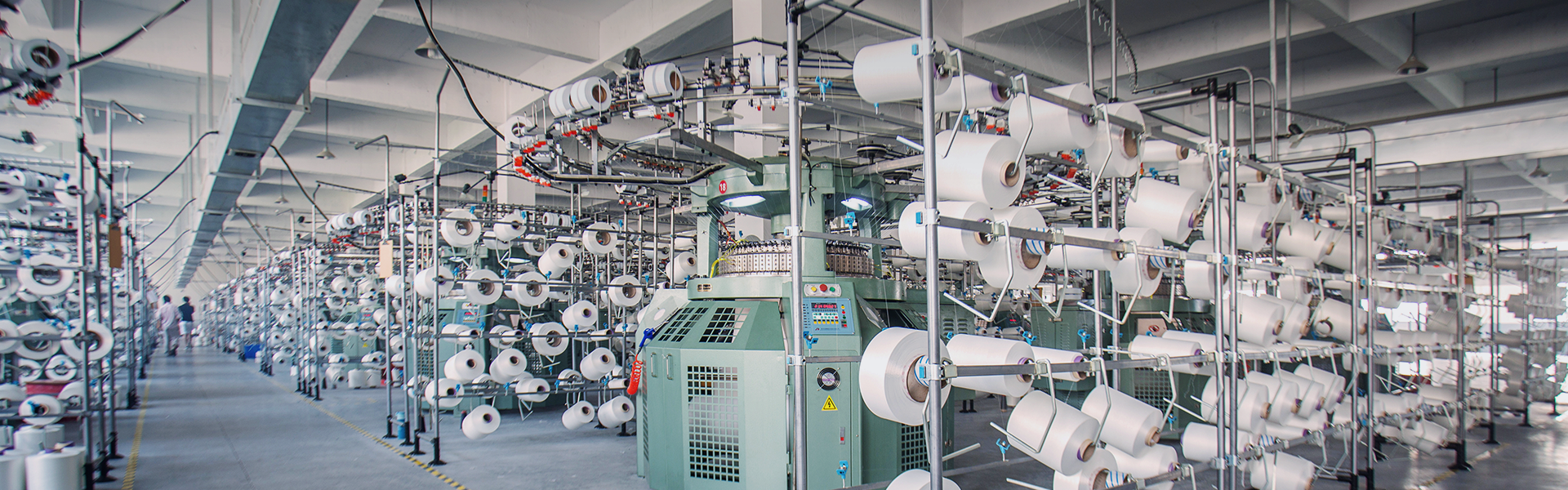••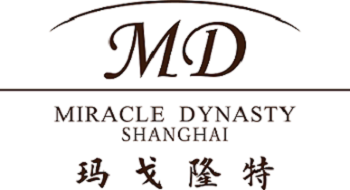+
•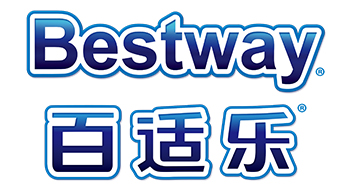+
•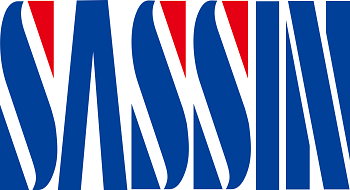+
•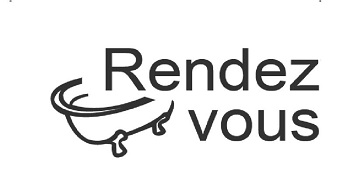+
•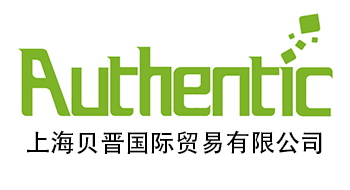+
•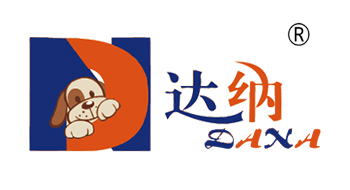+
•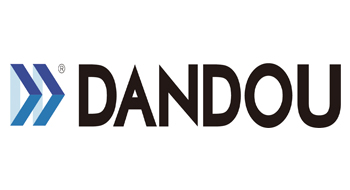+
•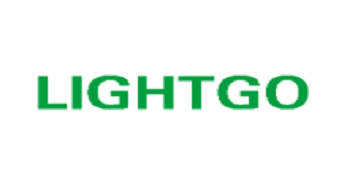+
•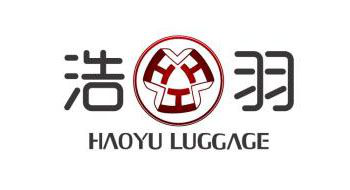+
•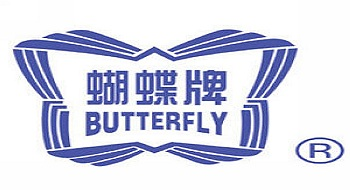+
•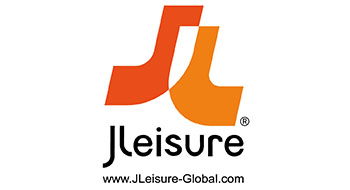+
•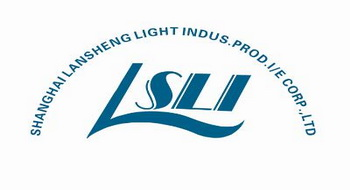+
•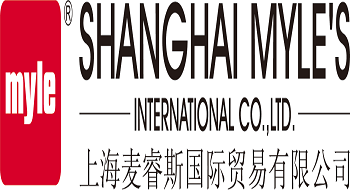+
•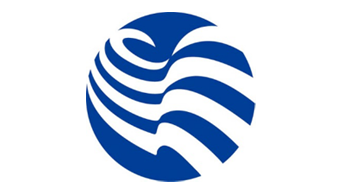+
•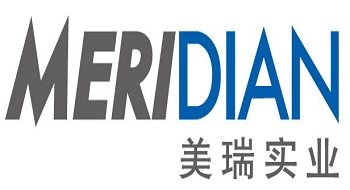+
•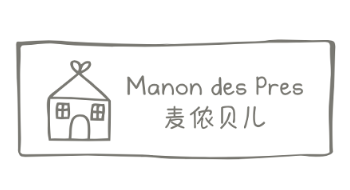+
•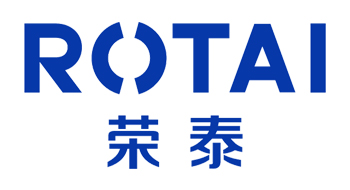+
•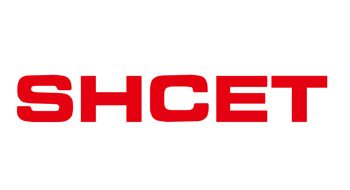+
•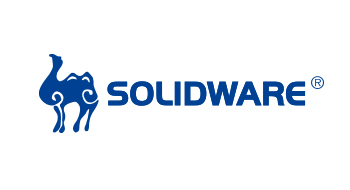+
•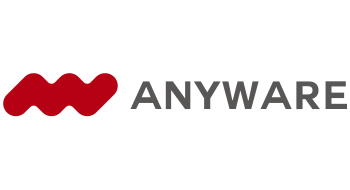+
•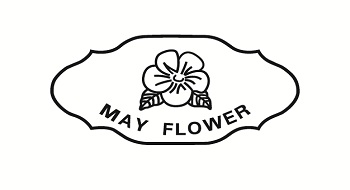+
•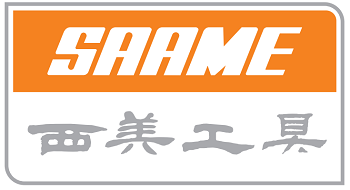+
•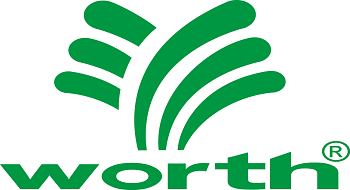+
•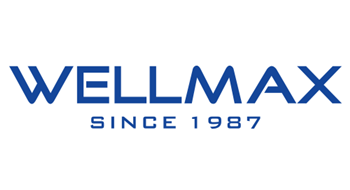+
•+
•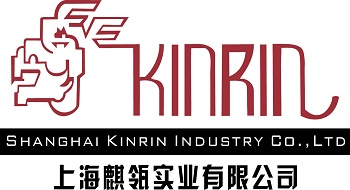+
•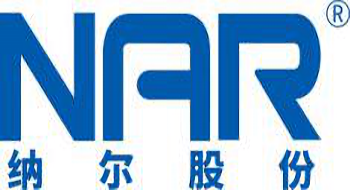+
•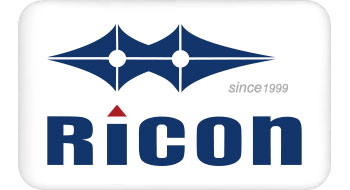+
•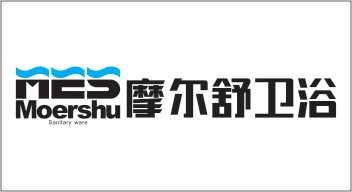+
•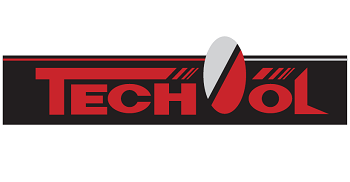+
•+
•+
•+
•+
•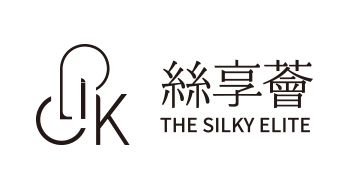+
•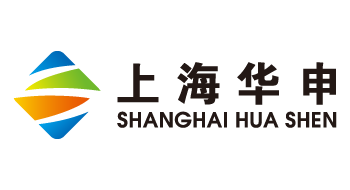+
•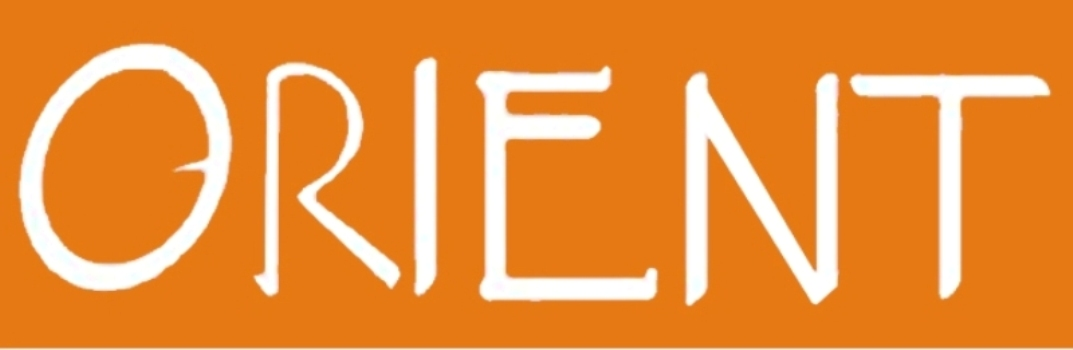+
•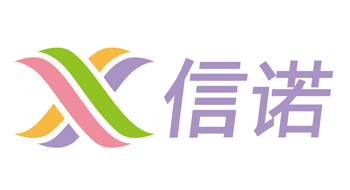+
•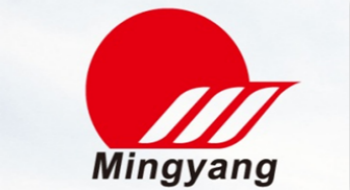+
•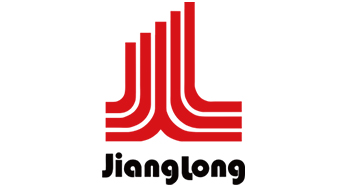+
•+
•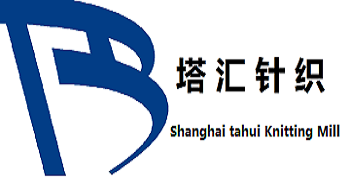+
•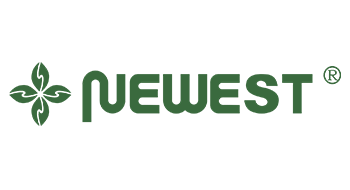+
•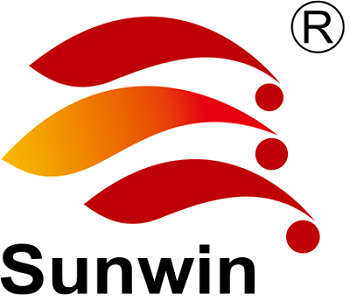+
•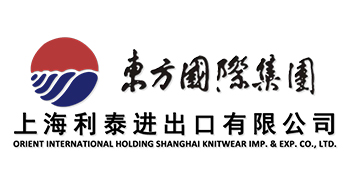+
•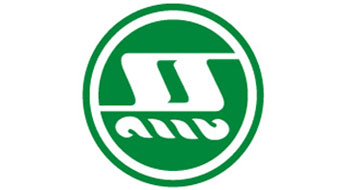+
•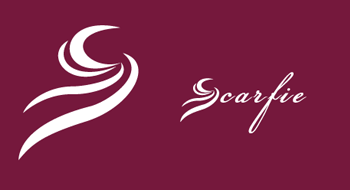+
•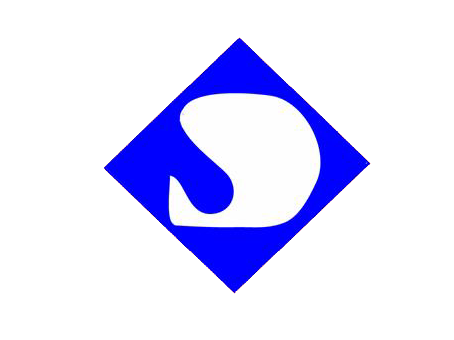+
•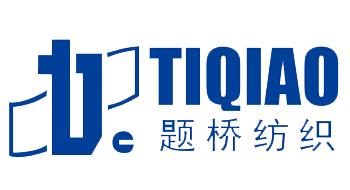+
•+
•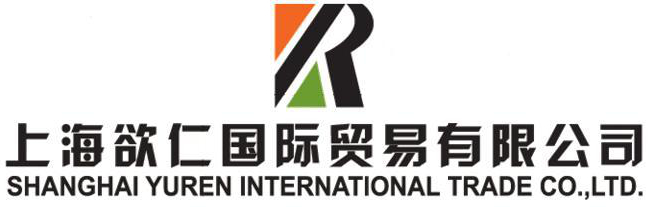+
•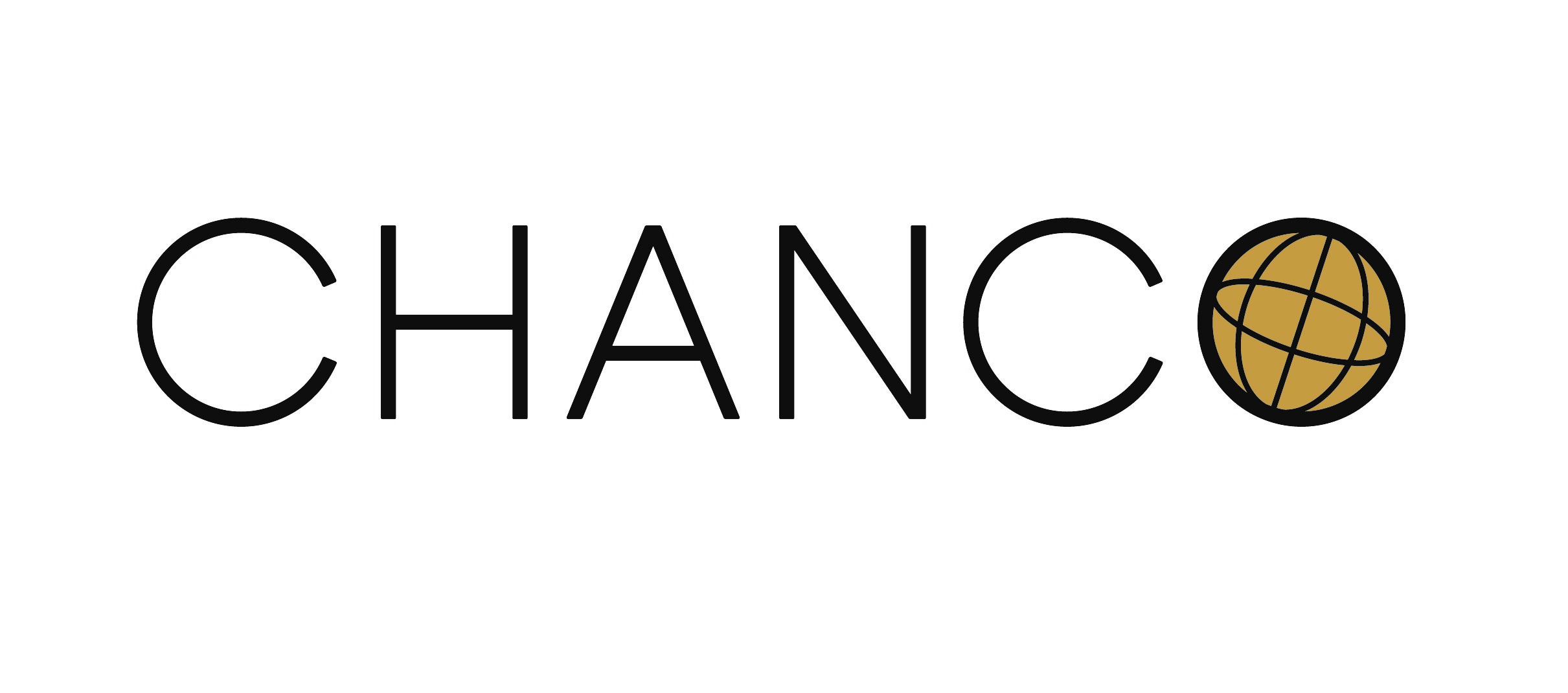+
•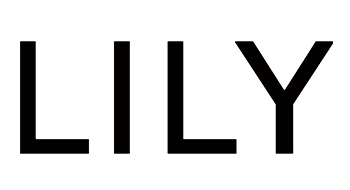+
•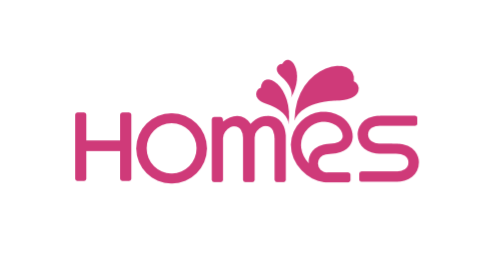+
•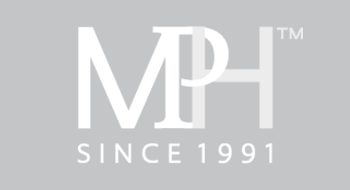+
•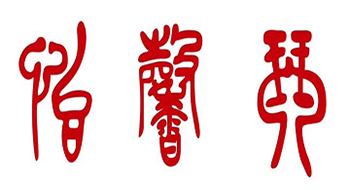+
•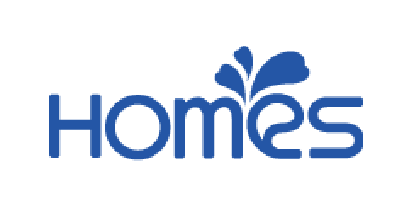+
•+
•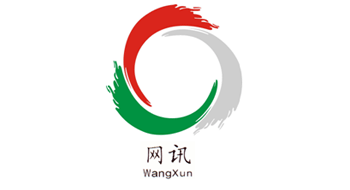+
•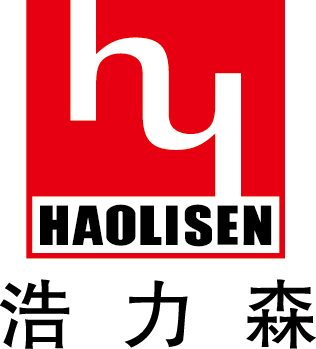+
•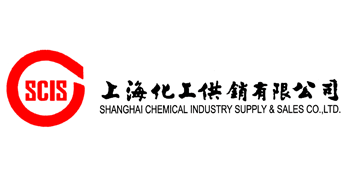+
•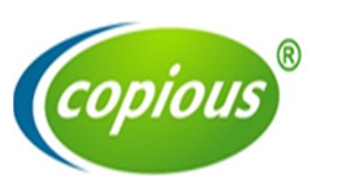+
•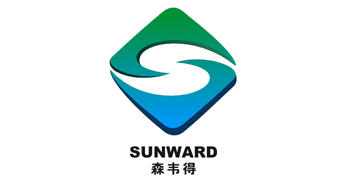+
•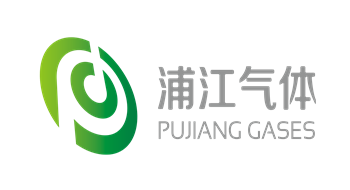+
•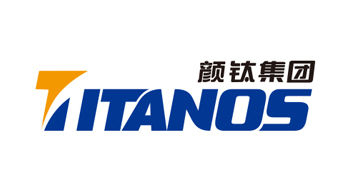+
•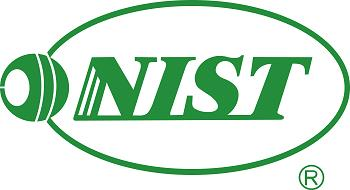+

• # 5•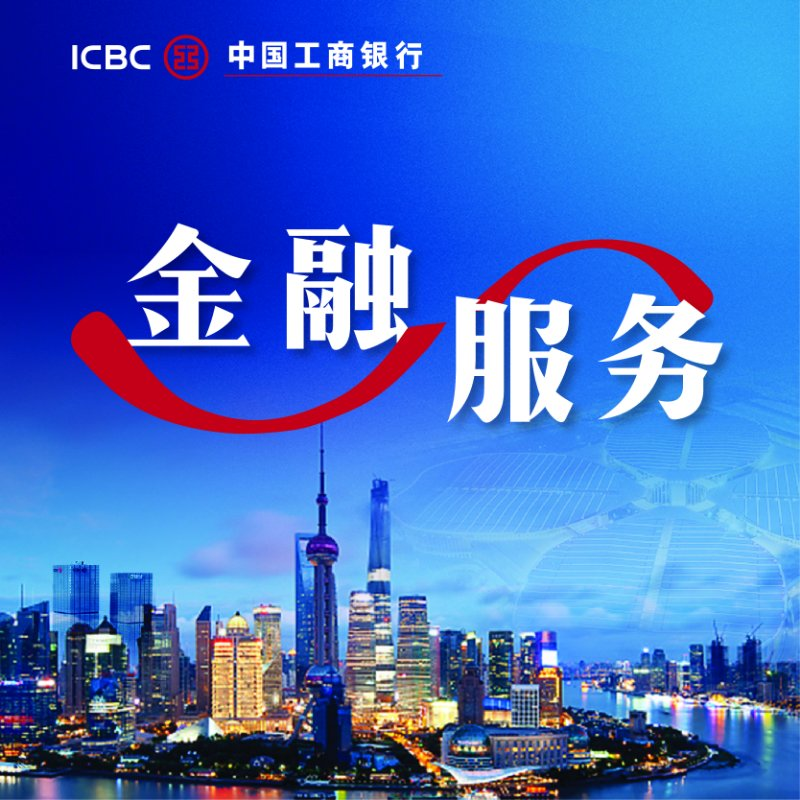•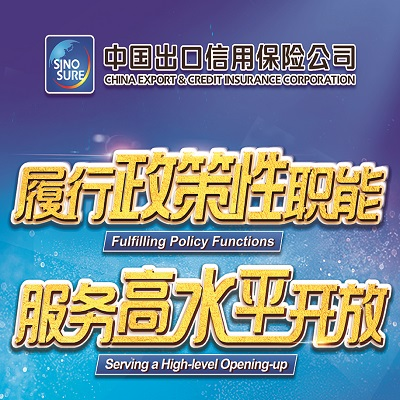•